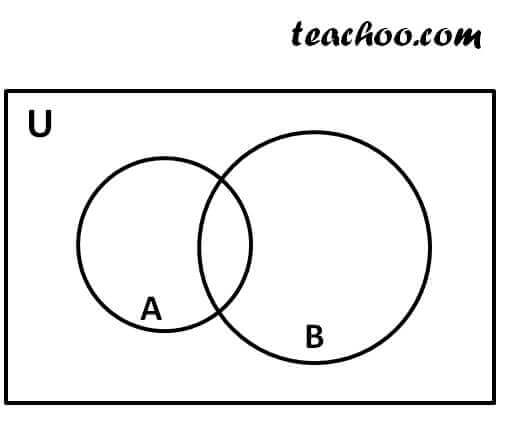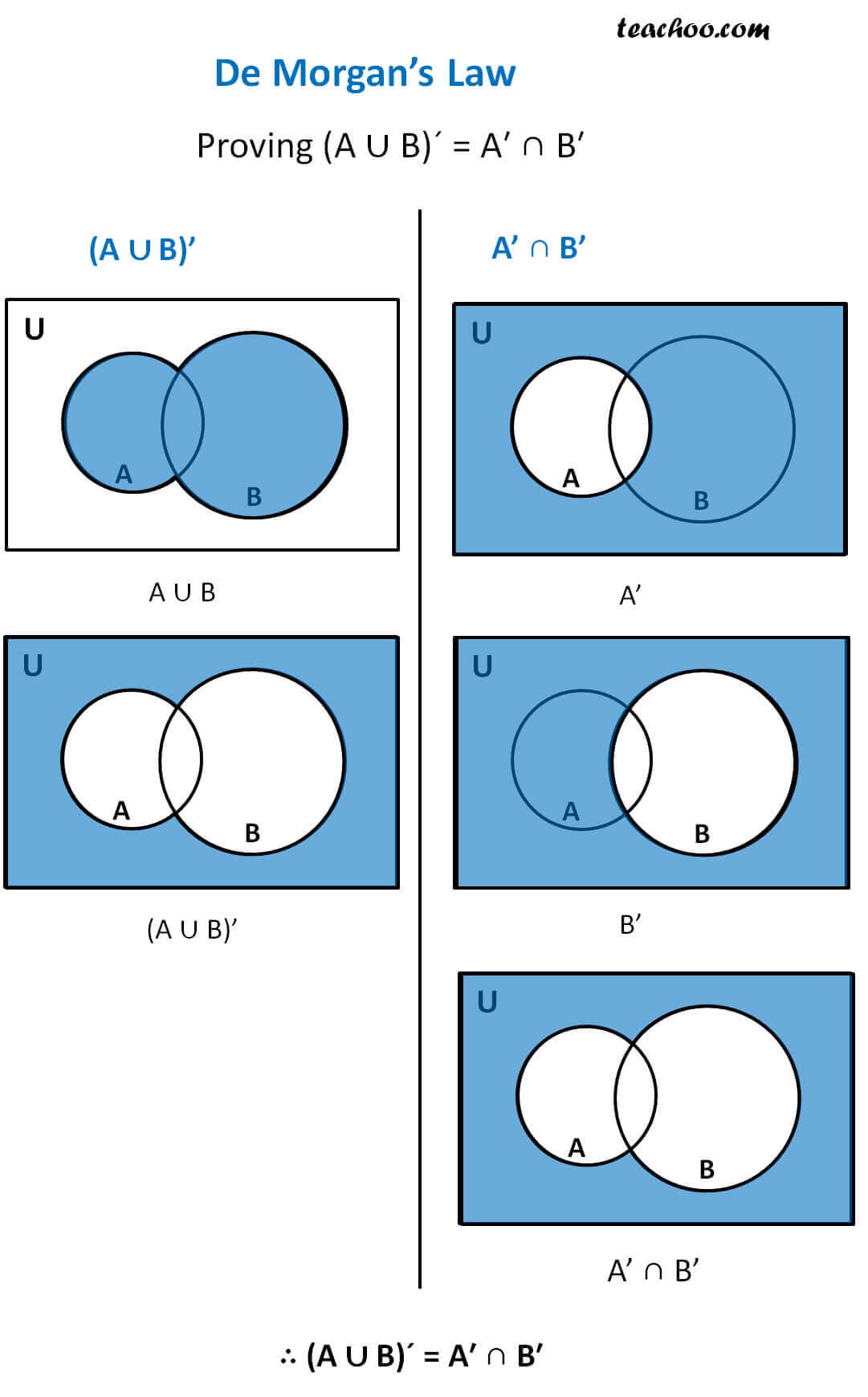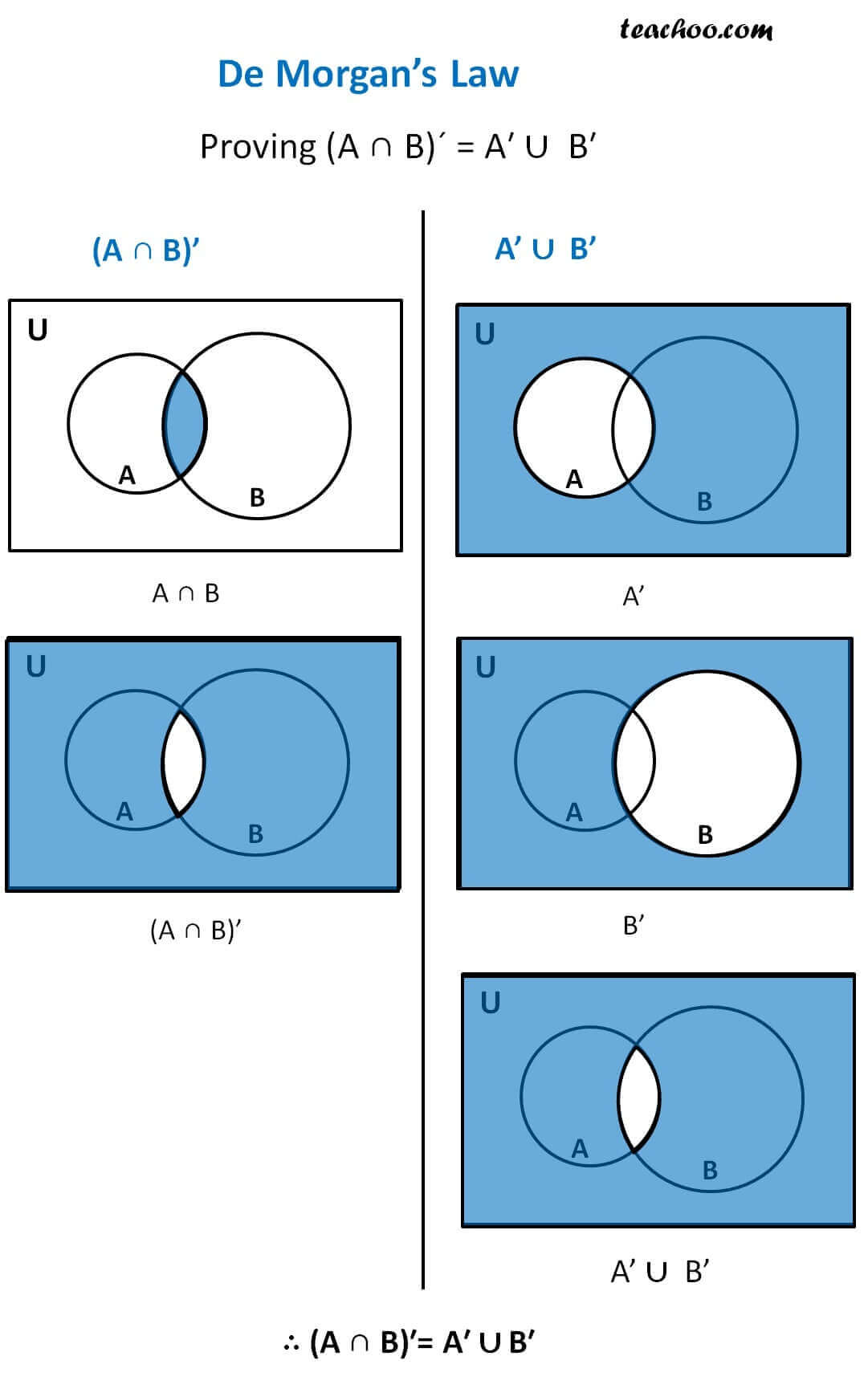De Morgan’s Law are based on complement of sets

1. (A ∪ B)´ = A′ ∩ B′
2. (A ∩ B)′ = A′ ∪ B′

Let us prove the law by Venn Diagrams

Let's take two sets A and B like## Proving (A ∪ B)´ = A′ ∩ B′## Proving (A ∩ B)′= A′ ∪ B′1. Chapter 1 Class 11 Sets (Term 1)
2. Concept wise
3. Complement of set